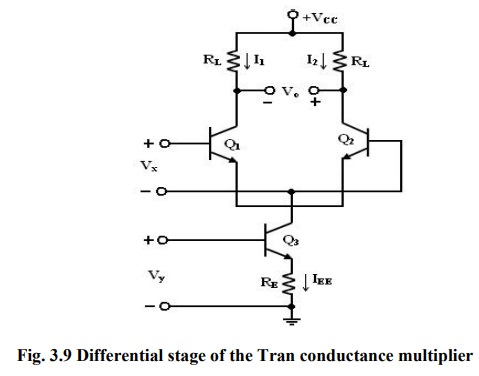Home | | Linear Integrated Circuits | Variable Transconductance Technique

# Variable Transconductance Technique

The variable transconductance technique makes use of the dependence characteristic of the transistor transconductance parameter on the emitter current bias applied.

Variable Transconductance Technique:·           The variable transconductance technique makes use of the dependence characteristic of the transistor transconductance parameter on the emitter current bias applied. A simple differential circuit arrangement depicting the principle is shown in figure.

·           The relationship between V0 and Vx . is given by V0 = gm RL VX where gm = IEE /VT is the transconductance of the stage.

·           Application of a second input Vy to the reference current source of the differential amplifier can vary gm.

·           Thus, if RE IEE >>VBE, the bias voltage Vy is related to IEE by the relation Vy = IEE RE.

·           Then, the overall voltage transfer expression is given by

V0 = gm RLVx = (Vy/VTRE)VxRL

= VxVy  RL/ VTRE

Tags : Analog Multiplier , Linear Integrated Circuits : Analog Multiplier and PLL
Study Material, Lecturing Notes, Assignment, Reference, Wiki description explanation, brief detail
Linear Integrated Circuits : Analog Multiplier and PLL : Variable Transconductance Technique | Analog Multiplier